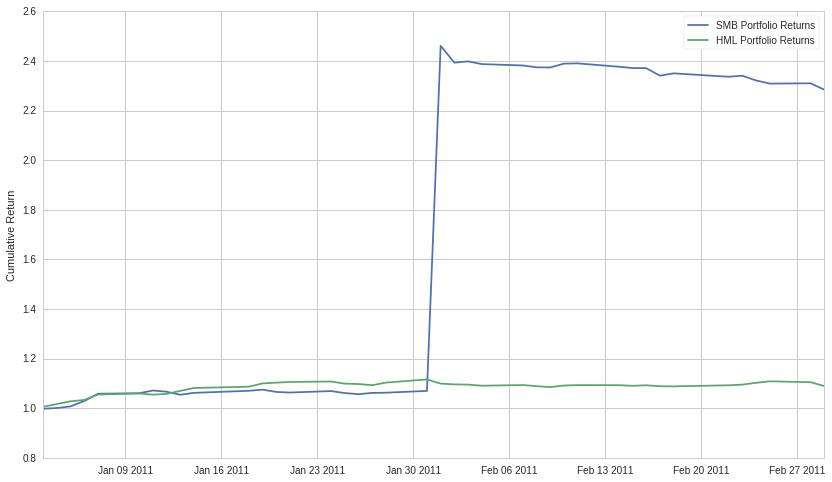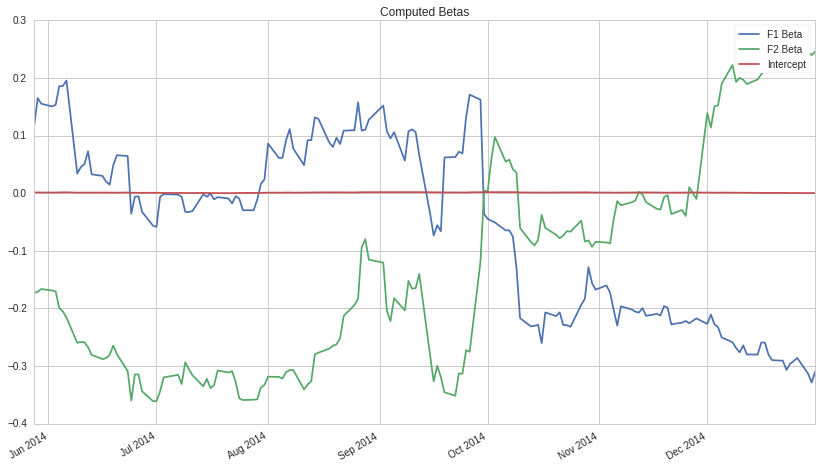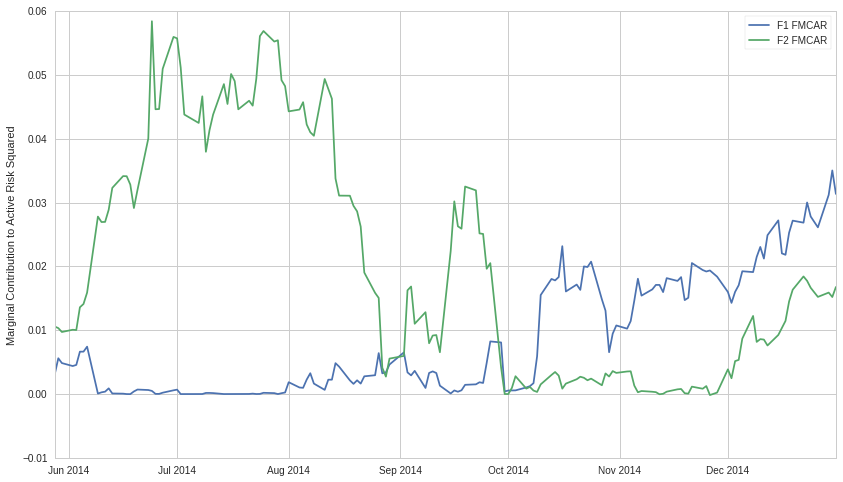Notebook

# Factor Risk Exposure¶

By Evgenia "Jenny" Nitishinskaya, Delaney Granizo-Mackenzie, and Maxwell Margenot.

Part of the Quantopian Lecture Series:

## DISCLAIMER:¶

As always, this analysis is based on historical data, and risk exposures estimated on historical data may or may not affect the exposures going forward. As such, computing the risk exposure of to a factor is not enough. You must put confidence bounds on that risk exposure, and determine whether the risk exposure can even be modeled reasonably. For more information on this, please see our other lectures, especially Instability of Parameter Estimates.

## Using Factor Models to Determine Risk Exposure¶

We can use factor models to analyze the sources of risks and returns in portfolios. Recall that a factor model expresses the returns as

$$R_i = a*i + b*{i1} F*1 + b*{i2} F*2 + \ldots + b*{iK} F_K + \epsilon_i$$

By modelling the historical returns, we can see how much of them is due to speculation on different factors and how much to asset-specific fluctuations ($\epsilon_p$). We can also examine what sources of risk the portfolio is exposed to.

In risk analysis, we often model active returns (returns relative to a benchmark) and active risk (standard deviation of active returns, also known as tracking error or tracking risk).

For instance, we can find a factor's marginal contribution to active risk squared (FMCAR). For factor $j$, this is

$$\text{FMCAR}_j = \frac{b*j^a \sum*{i=1}^K b_i^a Cov(F_j, F_i)}{(\text{Active risk})^2}$$

where $b_i^a$ is the portfolio's active exposure to factor $i$. This tells us how much risk we incur by being exposed to factor $j$, given all the other factors we're already exposed to.

Fundamental factor models are often used to evaluate portfolios because they correspond directly to investment choices (e.g. whether we invest in small-cap or large-cap stocks, etc.). Below, we construct a model to evaluate a single asset; for more information on the model construction, check out the fundamental factor models notebook.

We'll use the canonical Fama-French factors for this example, which are the returns of portfolios constructred based on fundamental factors.

## How many factors do you want?¶

In the Arbitrage Pricing Theory lecture we mention that for predictive models you want fewer parameters. However, this doesn't quite hold for risk exposure. Instead of trying to not overfit a predictive model, you are looking for any possible risk factor that could be influencing your returns. Therefore it's actually safer to estimate exposure to many many risk factors to see if any stick. Anything left over in our $\alpha$ is risk exposure that is currently unexplained by the selected factors. You want your strategy's return stream to be all alpha, and to be unexplained by as many parameters as possible. If you can show that your historical returns have little to no dependence on many factors, this is very positive. Certainly some unrelated risk factors might have spurious relationships over time in a large dataset, but those are not likely to be consistent.

## Setup¶

The first thing we do is compute a year's worth of factor returns.

### NOTE¶

The process for doing this is described in the Fundamental Factor Models lecture and uses pipeline. For more information please see that lecture.

In :
import numpy as np
import statsmodels.api as sm
import scipy.stats as stats
from statsmodels import regression
import matplotlib.pyplot as plt
import pandas as pd

In :
import numpy as np
from quantopian.pipeline import Pipeline
from quantopian.pipeline.data import Fundamentals
from quantopian.pipeline.data.builtin import USEquityPricing
from quantopian.pipeline.factors import CustomFactor, Returns

# Here's the raw data we need, everything else is derivative.

class MarketCap(CustomFactor):
# Here's the data we need for this factor
inputs = [Fundamentals.shares_outstanding, USEquityPricing.close]
# Only need the most recent values for both series
window_length = 1

def compute(self, today, assets, out, shares, close_price):
# Shares * price/share = total price = market cap
out[:] = shares * close_price

class BookToPrice(CustomFactor):
# pb = price to book, we'll need to take the reciprocal later
inputs = [Fundamentals.pb_ratio]
window_length = 1

def compute(self, today, assets, out, pb):
out[:] = 1 / pb

def make_pipeline():
"""
Create and return our pipeline.

We break this piece of logic out into its own function to make it easier to
test and modify in isolation.

In particular, this function can be copy/pasted into research and run by itself.
"""
pipe = Pipeline()

# Add our factors to the pipeline
market_cap = MarketCap()
# Raw market cap and book to price data gets fed in here
book_to_price = BookToPrice()

# We also get daily returns
returns = Returns(inputs=[USEquityPricing.close], window_length=2)

# We compute a daily rank of both factors, this is used in the next step,
# which is computing portfolio membership.
market_cap_rank = market_cap.rank()

book_to_price_rank = book_to_price.rank()

# Build Filters representing the top and bottom 1000 stocks by our combined ranking system.
biggest = market_cap_rank.top(1000)
smallest = market_cap_rank.bottom(1000)

highpb = book_to_price_rank.top(1000)
lowpb = book_to_price_rank.bottom(1000)

# Don't return anything not in this set, as we don't need it.
pipe.set_screen(biggest | smallest | highpb | lowpb)

# Add the boolean flags we computed to the output data

return pipe

pipe = make_pipeline()

In :
start_date = '2011-1-1'
end_date = '2011-3-1'

In :
from quantopian.research import run_pipeline
results = run_pipeline(pipe, start_date, end_date)

R_biggest = results[results.biggest]['returns'].groupby(level=0).mean()
R_smallest = results[results.smallest]['returns'].groupby(level=0).mean()

R_highpb = results[results.highpb]['returns'].groupby(level=0).mean()
R_lowpb = results[results.lowpb]['returns'].groupby(level=0).mean()

SMB = R_smallest - R_biggest
HML = R_highpb - R_lowpb


How did each factor do over 2014?

In :
SMB_CUM = np.cumprod(SMB+1)
HML_CUM = np.cumprod(HML+1)

plt.plot(SMB_CUM.index, SMB_CUM.values)
plt.plot(HML_CUM.index, HML_CUM.values)
plt.ylabel('Cumulative Return')
plt.legend(['SMB Portfolio Returns', 'HML Portfolio Returns']);### Computing Risk Exposure¶

Now we can determine how exposed another return stream is to each of these factors. We can do this by running static or rolling linear regressions between our return stream and the factor portfolio returns. First we'll compute the active returns (returns - benchmark) of some random asset and then model that asset as a linear combination of our two factors. The more a factor contributes to the active returns, the more exposed the active returns are to that factor.

In :
# Get returns data for our portfolio
portfolio = get_pricing(['MSFT', 'AAPL', 'YHOO', 'FB', 'TSLA'],
fields='price', start_date=start_date, end_date=end_date).pct_change()[1:]
R = np.mean(portfolio, axis=1)

bench = get_pricing('SPY', fields='price', start_date=start_date, end_date=end_date).pct_change()[1:]

# The excess returns of our active management, in this case just holding a portfolio of our one asset
active = R - bench

# Define a constant to compute intercept
constant = pd.TimeSeries(np.ones(len(active.index)), index=active.index)

df = pd.DataFrame({'R': active,
'F1': SMB,
'F2': HML,
'Constant': constant})
df = df.dropna()

/usr/local/lib/python2.7/dist-packages/ipykernel_launcher.py:13: FutureWarning: TimeSeries is deprecated. Please use Series
del sys.path

In :
# Perform linear regression to get the coefficients in the model
b1, b2 = regression.linear_model.OLS(df['R'], df[['F1', 'F2']]).fit().params

# Print the coefficients from the linear regression
print 'Sensitivities of active returns to factors:\nSMB: %f\nHML: %f' %  (b1, b2)

Sensitivities of active returns to factors:
SMB: -0.066887
HML: -0.046026


Using the formula from the start of the notebook, we can compute the factors' marginal contributions to active risk squared:

In :
F1 = df['F1']
F2 = df['F2']
cov = np.cov(F1, F2)
ar_squared = (active.std())**2
fmcar1 = (b1*(b2*cov[0,1] + b1*cov[0,0]))/ar_squared
fmcar2 = (b2*(b1*cov[0,1] + b2*cov[1,1]))/ar_squared
print 'SMB Risk Contribution:', fmcar1
print 'HML Risk Contribution:', fmcar2

SMB Risk Contribution: 0.00159645465913
HML Risk Contribution: 0.000973034746847


The rest of the risk can be attributed to active specific risk, i.e. factors that we did not take into account or the asset's idiosyncratic risk.

However, as usual we will look at how the exposure to these factors changes over time. As we lose a tremendous amount of information by just looking at one data point. Let's look at what happens if we run a rolling regression over time.

In :
# Compute the rolling betas
model = pd.stats.ols.MovingOLS(y = df['R'], x=df[['F1', 'F2']],
window_type='rolling',
window=100)
rolling_parameter_estimates = model.beta
rolling_parameter_estimates.plot();
plt.title('Computed Betas');
plt.legend(['F1 Beta', 'F2 Beta', 'Intercept']);

/usr/local/lib/python2.7/dist-packages/IPython/core/interactiveshell.py:2882: FutureWarning: The pandas.stats.ols module is deprecated and will be removed in a future version. We refer to external packages like statsmodels, see some examples here: http://statsmodels.sourceforge.net/stable/regression.html
exec(code_obj, self.user_global_ns, self.user_ns)Now we'll look at FMCAR as it changes over time.

In :
# Remove the first 99, which are all NaN for each case

# Compute covariances
covariances = pd.rolling_cov(df[['F1', 'F2']], window=100)[99:]
# Compute active risk squared
active_risk_squared = pd.rolling_std(active, window = 100)[99:]**2
# Compute betas
betas = rolling_parameter_estimates[['F1', 'F2']]

# Set up empty dataframe
FMCAR = pd.DataFrame(index=betas.index, columns=betas.columns)

# For each factor
for factor in betas.columns:
# For each bar in our data
for t in betas.index:
# Compute the sum of the betas and covariances
s = np.sum(betas.loc[t] * covariances[t][factor])
# Get the beta
b = betas.loc[t][factor]
# Get active risk squared
AR = active_risk_squared.loc[t]
# Put them all together to estimate FMCAR on that date
FMCAR[factor][t] = b * s / AR

/usr/local/lib/python2.7/dist-packages/ipykernel_launcher.py:4: FutureWarning: pd.rolling_cov is deprecated for DataFrame and will be removed in a future version, replace with
DataFrame.rolling(window=100).cov(other=<DataFrame>,pairwise=True)
after removing the cwd from sys.path.
/usr/local/lib/python2.7/dist-packages/ipykernel_launcher.py:6: FutureWarning: pd.rolling_std is deprecated for Series and will be removed in a future version, replace with
Series.rolling(window=100,center=False).std()



Let's plot this.

In :
plt.plot(FMCAR['F1'].index, FMCAR['F1'].values)
plt.plot(FMCAR['F2'].index, FMCAR['F2'].values)

plt.ylabel('Marginal Contribution to Active Risk Squared')
plt.legend(['F1 FMCAR', 'F2 FMCAR']);### Problems with using this data¶

Whereas it may be interesting to know how a portfolio was exposed to certain factors historically, it is really only useful if we can make predictions about how it will be exposed to risk factors in the future. It's not always a safe assumption to say that future exposure will be the current exposure. As you saw the exposure varies quite a bit, so taking the average is dangerous. We could put confidence intervals around that average, but that would only work if the distribution of exposures were normal or well behaved. Let's check using our old buddy, the Jarque-Bera test.

In :
from statsmodels.stats.stattools import jarque_bera
_, pvalue1, _, _ = jarque_bera(FMCAR['F1'].dropna().values)
_, pvalue2, _, _ = jarque_bera(FMCAR['F2'].dropna().values)

print 'p-value F1_FMCAR is normally distributed', pvalue1
print 'p-value F2_FMCAR is normally distributed', pvalue2

p-value F1_FMCAR is normally distributed 3.45995832983e-05
p-value F2_FMCAR is normally distributed 0.000393310890239


The p-values are below our default cutoff of 0.05. We can't even put good confidence intervals on the risk exposure of the asset without extra effort, so making any statement about exposure in the future is very difficult right now. Any hedge we took out to cancel the exposure to one of the factors might be way over or under hedged.

We are trying to predict future exposure, and predicting the future is incredibly difficult. One must be very careful with statistical methods to ensure that false predictions are not made.

# Factor and tracking portfolios¶

We can use factor and tracking portfolios to tweak a portfolio's sensitivities to different sources of risk.

A factor portfolio has a sensitivity of 1 to a particular factor and 0 to all other factors. In other words, it represents the risk of that one factor. We can add a factor portfolio to a larger portfolio to adjust its exposure to that factor.

A similar concept is a tracking portfolio, which is constructed to have the same factor sensitivities as a benchmark or other portfolio. Like a factor portfolio, this allows us to either speculate on or hedge out the risks associated with that benchmark or portfolio. For instance, we regularly hedge out the market, because we care about how our portfolio performs relative to the market, and we don't want to be subject to the market's fluctuations.

To construct a factor or tracking portfolio, we need the factor sensitivities of what we want to track. We already know what these are in the former case, but we need to compute them in the latter using usual factor model methods. Then, we pick some $K+1$ assets (where $K$ is the number of factors we're considering) and solve for the weights of the assets in the portfolio.

## Portfolio Exposure¶

The portfolio exposure can be computed directly from the return stream, or as the weighted average of all the assets held.

## Example¶

Say we have two factors $F_1$ and $F_2$, and a benchmark with sensitivities of 1 and 1.1 to the factors, respectively. We identify 3 securities $x_1, x_2, x*3$ that we would like to use in composing a portfolio that tracks the benchmark, whose sensitivities are $b*{11} = 0.7$, $b*{12} = 1.1$, $b*{21} = 0.1$, $b*{22} = 0.5$, $b*{31} = 1.5$, $b_{32} = 1.3$. We would like to compute weights $w_1$, $w_2$, $w_3$ so that our tracking portfolio is

$$P = w_1 x_1 + w_2 x_2 + w_3 x_3$$

We want our portfolio sensitivities to match the benchmark:

$$w*1 b*{11} + w*2 b*{21} + w*3 b*{31} = 1$$ $$w*1 b*{12} + w*2 b*{22} + w*3 b*{32} = 1.1$$

Also, the weights need to sum to 1:

$$w_1 + w_2 + w_3 = 1$$

Solving this system of 3 linear equations, we find that $w_1 = 1/3$, $w_2 = 1/6$, and $w_3 = 1/2$. Putting the securities together into a portfolio using these weights, we obtain a portfolio with the same risk profile as the benchmark.

## How to Use Risk Exposure Models¶

Once we know our risk exposures, we can do a few things. We can not enter into positions that have high exposures to certain factors, or we can hedge our positions to try to neutralize the exposure.

### Risk Management¶

Often times funds will have a layer of protection over their traders/algorithms. This layer of protection takes in the trades that the fund wants to make, then computes the exposure of the new portfolio, and checks to make sure they're within pre-defined ranges. If they are not, it does not place the trade and files a warning.

### Hedging¶

Another method of dealing with exposure is to take out hedges. You can determine, for example, your exposure to each sector of the market. You can then take out a hedge if a particular sector seems to affect your returns too much. For more information on hedging, please see our Beta Hedging lecture. Good algorithms will have built-in hedging logic that ensures they are never over-exposed.

This presentation is for informational purposes only and does not constitute an offer to sell, a solicitation to buy, or a recommendation for any security; nor does it constitute an offer to provide investment advisory or other services by Quantopian, Inc. ("Quantopian"). Nothing contained herein constitutes investment advice or offers any opinion with respect to the suitability of any security, and any views expressed herein should not be taken as advice to buy, sell, or hold any security or as an endorsement of any security or company. In preparing the information contained herein, Quantopian, Inc. has not taken into account the investment needs, objectives, and financial circumstances of any particular investor. Any views expressed and data illustrated herein were prepared based upon information, believed to be reliable, available to Quantopian, Inc. at the time of publication. Quantopian makes no guarantees as to their accuracy or completeness. All information is subject to change and may quickly become unreliable for various reasons, including changes in market conditions or economic circumstances.